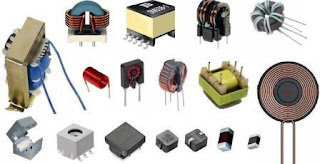# What is Inductor - Basic idea

An inductor is an electrical component that stores energy in a magnetic field when electric current flows through it. It can be used in a variety of e

# What is Inductor - Basic idea

An inductor is an electrical component that stores energy in a magnetic field when electric current flows through it. It can be used in a variety of electrical circuits and devices, such as filters, transformers, and oscillators.
The amount of energy stored in an inductor is determined by its inductance, which is measured in units of henries (H). Inductors are often represented in circuit diagrams by the symbol of a series of loops.

## Type of Inductor:There are several types of inductors,

## Air-core inductors:

These inductors do not have a core made of a magnetic material. They are simple to construct and have a relatively high inductance per unit length, but their inductance is not as stable as other types.

## Iron-core inductors:

These inductors have a core made of a magnetic material such as iron. They have a higher inductance than air-core inductors, but their inductance is also less stable due to the magnetic properties of the core material changing with temperature.

## Ferrite-core inductors:

These inductors have a core made of a ferrite material. Ferrite is a type of ceramic material with high magnetic permeability. Ferrite-core inductors have high inductance and are relatively stable over a wide range of temperatures.

## Toroidal inductors:

These inductors are shaped like a torus (ring) and have a core made of a magnetic material. They have a high inductance per unit volume and are relatively easy to wind.

## Spiral inductors:

These inductors are made by winding a wire in a spiral pattern around a core. They can be made in different shapes and sizes, and have a high inductance per unit length.

## Multi-layer inductors:

These inductors are made by winding a wire around a core and then stacking multiple layers of wire on top of each other. They have high inductance per unit volume and are commonly used in power supplies.

## Variable inductors:

These inductors have a way to adjust the inductance, for example by moving a ferrite core, or by adjusting the number of turns of wire. They are used in RF circuit design, tuners and matching network.
Labels: